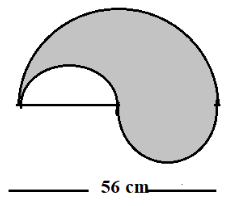# The boundary of the shaded region in the adjoining figure is1. A
200 mm
2. B
196 mm
3. C
186 mm
4. D
176 mm

Fill Out the Form for Expert Academic Guidance!l

+91

Live ClassesBooksTest SeriesSelf Learning

Verify OTP Code (required)

### Solution:

In the figure, there are three semicircles, where the diameter of one semicircle is 56 cm.
The radius of a semicircle is  .
The circumference of the semicircle is,  .
Similarly, for a semi-circle which has diameter 28 cm, the radius is  .

For the third semicircle, it is also 44 mm.
So, the boundary of the shaded region is,

Hence, the correct option is 4.

## Related content

 Area of Square Area of Isosceles Triangle Pythagoras Theorem Triangle Formula Perimeter of Triangle Formula Area Formulae Volume of Cone Formula Matrices and Determinants_mathematics Critical Points Solved Examples Type of relations_mathematics+91

Live ClassesBooksTest SeriesSelf Learning

Verify OTP Code (required)